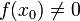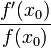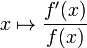# Logarithmic derivative

## Definition

### At a point

Suppose$f$ is a function and$x_0$ is a point in the interior of the domain of$f$ such that the derivative$f'(x_0)$ exists, and$f(x_0) \ne 0$ (note that$f'(x_0)$ may be zero or nonzero). The logarithmic derivative of$f$ at$x_0$ is defined as:$\! \frac{f'(x_0)}{f(x_0)}$

Alternatively, it can be defined as:$\! \frac{d}{dx}(\ln|f(x)|)|_{x = x_0}$

### As a function

Suppose$f$ is a function of one variable. The logarithmic derivative of$f$ is the function:$\! x \mapsto \frac{f'(x)}{f(x)}$

Alternatively, it is the function:$\! x \mapsto \frac{d}{dx}(\ln|f(x)|)$

The domain of this function is precisely the set of points$x$ for which$f(x) \ne 0$ and the derivative$f'$ exists.

MORE ON THE WAY THIS DEFINITION OR FACT IS PRESENTED: We first present the version that deals with a specific point (typically with a$\{ \}_0$ subscript) in the domain of the relevant functions, and then discuss the version that deals with a point that is free to move in the domain, by dropping the subscript. Why do we do this?
The purpose of the specific point version is to emphasize that the point is fixed for the duration of the definition, i.e., it does not move around while we are defining the construct or applying the fact. However, the definition or fact applies not just for a single point but for all points satisfying certain criteria, and thus we can get further interesting perspectives on it by varying the point we are considering. This is the purpose of the second, generic point version.
DIVISION BY ZERO: The formulation of this definition or fact involves a denominator that could a priori be zero. To avoid this, we stipulate conditions in our formulation that exclude the points or situations where the denominator is zero. It may or may not be possible to use concepts of limits to extend the definition somewhat to some of the excluded points.